# Kulraj Suri

Software Engineer / Quant Dev

# Julia Basics

1. What is Julia?

• Developed by MIT
• Fully open source
• Convenient syntax for building math constructs like vectors, matrices, etc
• Very fast

2. Getting Started

1. Run Julia in the cloud:

2. Run Julia locally (recommended):

• Faster and more flexible
• Requires some experience with command line

Install Julia:

Open the Julia application:

• You will be presented with a Julia command line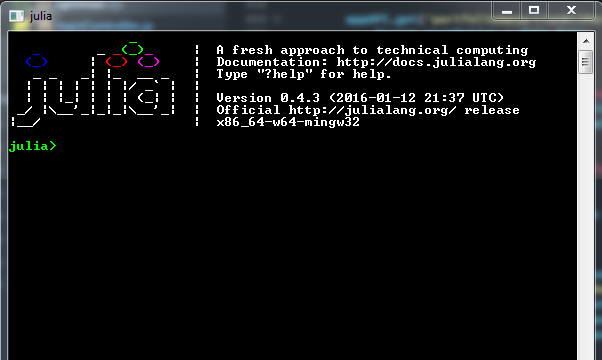Create a Julia .jl file:

• Create and save a .jl file in a text editor

Open a Julia .jl file:

• cd to the directory where your .jl file is located – remember to use “ \\ ”
• Use “include” to run the .jl file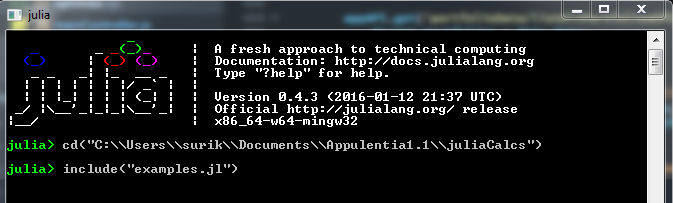3. Basics

Types

• Integers: Int64, e.g. -45
• Real numbers: Float64, e.g. 1.256
• Booleans: Bool, true or false
• Strings: ASCIIString, e.g. “Hello World”
• Arrays: Array(Int64, 3) = 3 element array with Int64 values, e.g. [45, 3453, -23]

+, -, *, / OperatorsExponential’s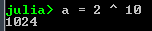Boolean Expressions

• Evaluate to true or false
• Use the operators: == , != , < , > , <= , >=Random Numbers

• Generate a random number between 0 and 1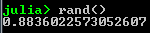4. Variables

• Assignments use the  = operator
• E.g. value = 5.0
• E.g. name = “Bob”5. Control Flow (if statements, for loops)

If Else Statements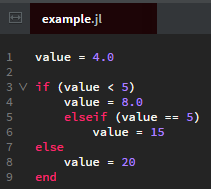• We see the result when we run this file in Julia:For Loops• We see the result when we run this file in Julia:While Loops• We see the result when we run this file in Julia: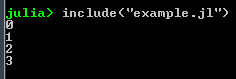6. Functions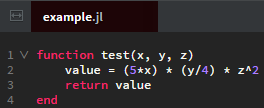7. Arrays and Matrices

Arrays

• Arrays can be initialised directly:• Or initialised empty: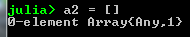• Arrays like this can be initialised with a type:• Ranges can be used to create arrays: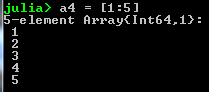• Arrays can also be generated from comprehensions: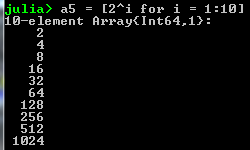• We can access elements of the array: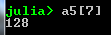• Arrays can be any type, so arrays of arrays can be created:• We can push to arrays like so:Matrices

• Matrices in Julia are represented by 2D arrays
• To create a 2×3 matrix:• Semicolons delimit rows; spaces delimit entries in a row
• size(A) returns the size of A as a pair (n rows x m columns):• Row vectors are 1xn matrices:Indexing and Slicing

• Aij is found with A[ i, j ]:• Ranges can also be used:• : selects all elements along that dimension
• A[ : , 3 ] selects the third column: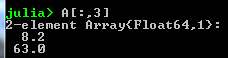• A[ 2, : ] selects the second row: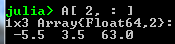• A[ : ] returns the columns of A stacked as a vector:Common Matrices

• zeros( n, m ):• ones( n, m ):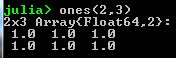• eye(n):• diag(x) where x is a vector:• Random nxm matrix:• A transposed is written “ A’ “:• Adding and subtraction of matrices, e.g.is written:Matrix Scalar Operations

• All matrix scalar operations ( +, -, * ) apply element wiseIs equivalent to:• Scalar multiplication:Is equivalent to: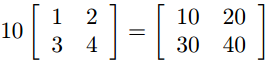Matrix Vector Multiplication

• The * operator is used for matrix vector multiplication
• For e.g.Is written: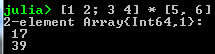Matrix Multiplication

• The * operator is used for matrix multiplicationIs written:8. Packages

• List all available packages:• Install one package (e.g. Calculus) and all it’s dependencies:• To list all installed packages:• To update all packages to their newest version:• To use a package:• This will import all functions of that package into the current namespace, so that it is possible to call: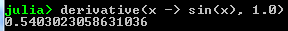• … without specifying the package it is included in
• You can also specify which package the function is called from:• … using ‘import’ is especially useful if there are conflicts in function/type-names between packages
• For example, the plotting packages ‘Winston’ and ‘Gadfly’ both use the ‘plot’ function
• This can be prevented by using ‘import’, as follows: